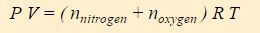Chemistry

# What is Dalton’s Law?

Dalton’s Law:

The pressure of a mixture of gases is equal to the sum of the partial pressures of the component gases.

Concepts

One of the important predictions made by Avogadro is that the identity of a gas is unimportant in determining the P-V-T properties of the gas. This behavior means that a gas mixture behaves in exactly the same fashion as a pure gas. (Indeed, early scientists such as Robert Boyle studying the properties of gases performed their experiments using gas mixtures, most notably air, rather than pure gases.)

The ideal gas law, P V = n R T ,

predicts how the pressure, volume, and temperature of a gas depend upon the number of moles of the gas. The number of moles, n, is the total moles of all the gas-phase species.

Air, for example, is composed primarily of nitrogen and oxygen. In a given sample of air, the total number of moles is can be approximated as:This expression for n can be substituted into the ideal gas law to yieldAll molecules in the gas have access to the entire volume of the system, thus V is the same for both nitrogen and oxygen. Similarly, both compounds experience the same temperature. One can therefore split this expression of the ideal gas law into two terms, one for nitrogen and one for oxygen.The above equation is called Dalton’s Law of Partial Pressure, and it states that the pressure of a gas mixture is the sum of the partial pressures of the individual components of the gas mixture. P nitrogen is the partial pressure of the nitrogen and P oxygen is the partial pressure of oxygen.You’ll notice that the equations for the partial pressures are really just the ideal gas law, but the moles of the individual component (nitrogen or oxygen) are used instead of the total moles. Conceptually P nitrogen is the contribution nitrogen molecules make to the pressure and P oxygen is the contribution oxygen molecules make.